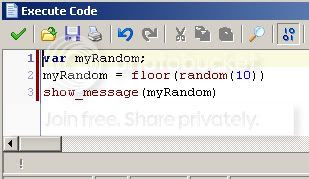Question:
How do I generate a random number within a range in Game Maker?The code above will create a whole random number between 0 and 9 for you. Why is that?

floor() is a function that rounds down what ever is the argument of it. random() creates a random number. random() uses the argument you put in and will never go over that number when generating a number.

So if you put in 10 as the argument for random, the random() function could generate numbers like 8.99, 9.4532, 9.99, but never 10. So when the floor() rounds the number down, the highest it can be is 9! Isn't that neat!

So if you wanted a number between 0 and 456, how would you do it? Wouldn't you put in this code?

floor(random(457));

Yep! We put in 457 as the argument because the random number will never go over 457, but will give you numbers that will always round down, with the highest being 456!

Try it out!

 Page updated: March 23rd, 2015 @ 6:21 AM Eastern Time